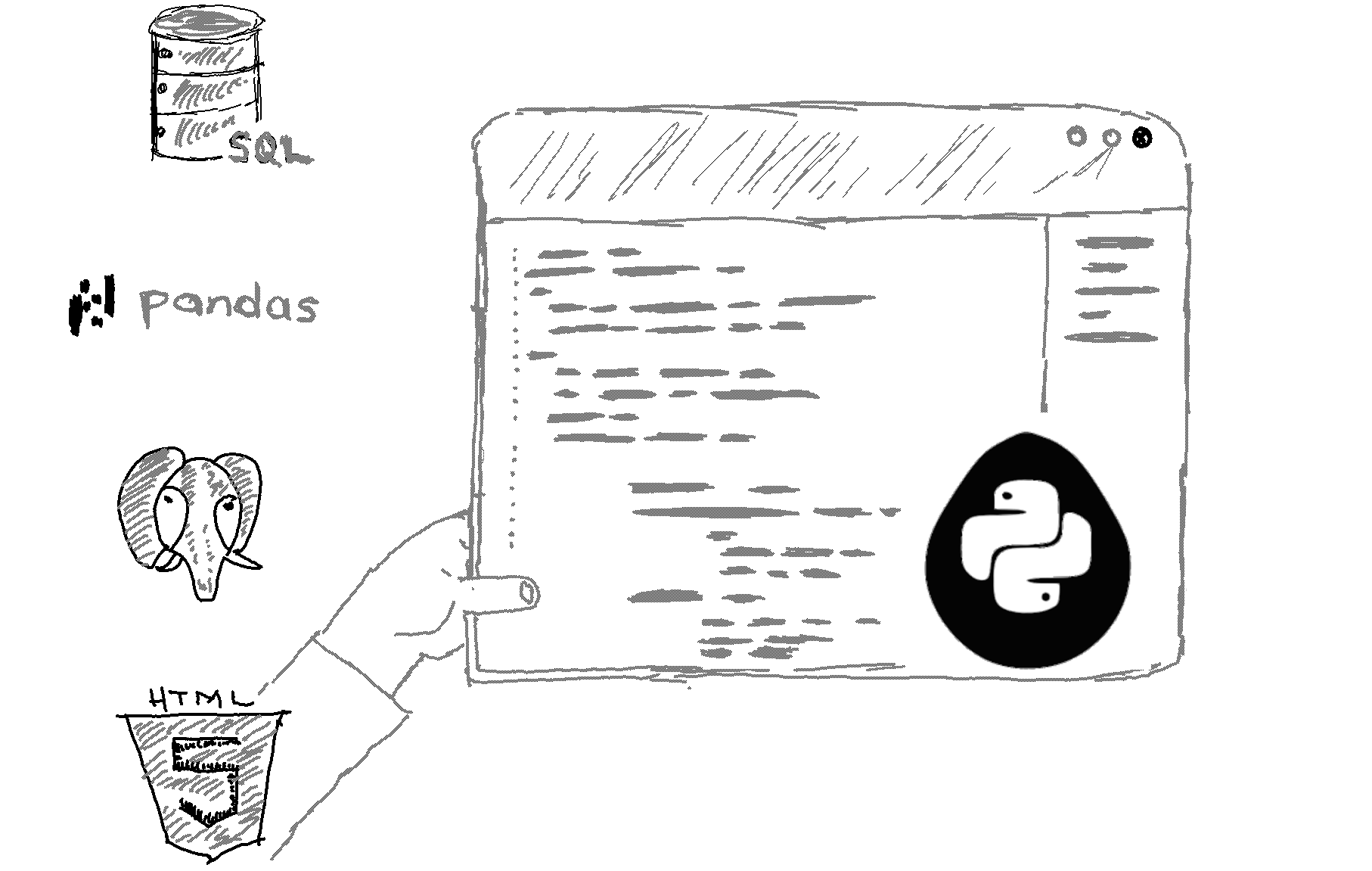Pandas.

That’s definitely the synonym of “Python for data analysis”.

Pandas is a powerful data analysis Python library that is built on top of numpy which is yet another library that lets you create 2D and even 3D arrays of data in Python. The pandas main object is called a dataframe. A dataframe is basically a 2D numpy array with rows and columns, that also has labels for columns and rows.

You can create data frames out of various input data formats such as CSV, JSON, Python dictionaries, etc. Once you have the dataframe loaded in Python, you can apply various data analysis and visualization functions to the dataframe and basically turn the dataframe data into valuable information. See how easy it is to create a pandas dataframe out of this CSV file.

`import pandas as pddf1 = pd.read_csv("C:/PythonHow/income_data.csv")print(df1)`

That code would generate the following output in Python:That’s how it looks on a basic Python shell. If you want a fancier look of the dataframe, you would want to use the Jupyter notebook to write and run your Python code. You will learn how to set up and use the Jupyter notebook in the next lesson of this tutorial, but for now, let’s just see how the same dataframe would look on a Jupyter notebook:Again, let’s focus on the code for now.

What we basically did is we imported the pandas dataframe and assigned the pd namespace to it for the sake of code abbreviation. Then we used the read_csv method of the pandas library to read a local CSV file as a dataframe. Lastly, we printed out the dataframe. If you want to understand how read_csv works, do some code introspection:

This will print out the help string for the read_csv method. Note that the header parameter was set to True by default. That means the method automatically detects and assigns the first row of the CSV file as the pandas dataframe header. If you didn’t have a header in your csv data, you would want to set the header parameter to None explicitly:

`df1 = pd.read_csv("C:/PythonHow/file1.csv", header=None)`

That’s no brainier that having a header is a good idea. You can refer to your columns when you want to extract specific columns of the dataframe or even portions of rows and columns.

You will learn how to slice your dataframe and calculate means of those slices in the next lesson.

Next Lecture